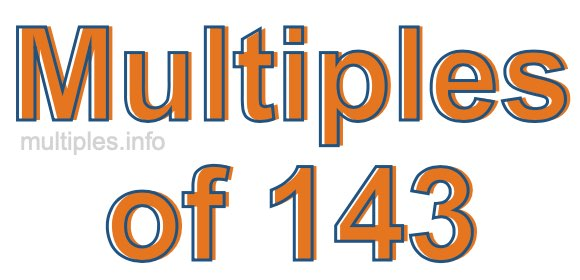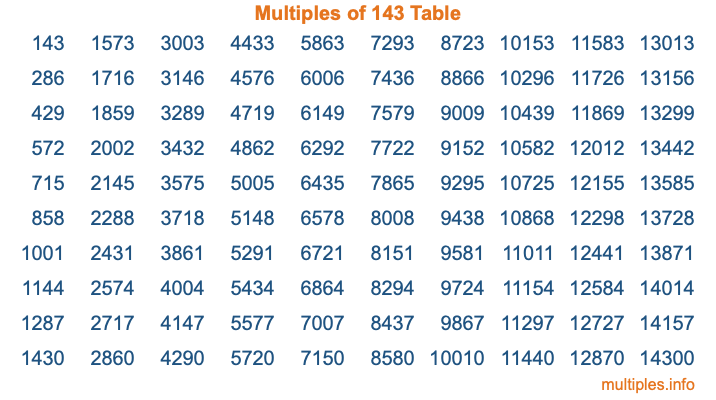Multiples of 143Welcome to the Multiples of 143 page. Here we will first teach you everything you will ever need to know about the multiples of 143, and then give you a study guide summary of everything we taught you to make sure you remember it all. Use this page to look up facts and learn information about the multiples of 143. This page will make you a multiples of one hundred forty-three expert!

Definition of Multiples of 143
Multiples of 143 are all the numbers that when divided by 143 equal an integer. Each of the multiples of 143 are called a multiple. A multiple of 143 is created by multiplying 143 by an integer.

Therefore, to create a list of multiples of 143, you start with 1 multiplied by 143, then 2 multiplied by 143, then 3 multiplied by 143, and so on for as long as you want. Thus, the list of the first five multiples of 143 is 143, 286, 429, 572, and 715. To see a larger list of multiples of 143, see the printable image of Multiples of 143 further down on this page. We also have a category where you can choose any nth multiple of 143.

Multiples of 143 Checker
The Multiples of 143 Checker below checks to see if any number of your choice is a multiple of 143. In other words, it checks to see if there is any number (integer) that when multiplied by 143 will equal your number. To do that, we divide your number by 143. If the the quotient is an integer, then your number is a multiple of 143.

Is  a multiple of 143?

Least Common Multiple of 143 and ...
A Least Common Multiple (LCM) is the lowest multiple that two or more numbers have in common. This is also called the smallest common multiple or lowest common multiple and is useful to know when you are adding our subtracting fractions. Enter one or more numbers below (143 is already entered) to find the LCM.

Check out our LCM Calculator if you need more details about the Least Common Multiple or if you need the LCM for different numbers for adding and subtraction fractions.

nth Multiple of 143
As we stated above, 143 is the first multiple of 143, 286 is the second multiple of 143, 429 is the third multiple of 143, and so on. Enter a number below to find the nth multiple of 143.

th multiple of 143

Multiples of 143 vs Factors of 143
143 is a multiple of 143 and a factor of 143, but that is where the similarities end. All postive multiples of 143 are 143 or greater than 143. All positive factors of 143 are 143 or less than 143.

Below is the beginning list of multiples of 143 and the factors of 143 so you can compare:

Multiples of 143: 143, 286, 429, 572, 715, etc.

Factors of 143: 1, 11, 13, 143

As you can see, the multiples of 143 are all the numbers that you can divide by 143 to get a whole number. The factors of 143, on the other hand, are all the whole numbers that you can multiply by another whole number to get 143.

It's also interesting to note that if a number (x) is a factor of 143, then 143 will also be a multiple of that number (x).

Multiples of 143 vs Divisors of 143
The divisors of 143 are all the integers that 143 can be divided by evenly. Below is a list of the divisors of 143.

Divisors of 143: 1, 11, 13, 143

The interesting thing to note here is that if you take any multiple of 143 and divide it by a divisor of 143, you will see that the quotient is an integer.

Multiples of 143 Table
Below is an image of the first 100 multiples of 143 in a table. The table is in chronological order, column by column. The first column has the first ten multiples of 143, the second column has the next ten multiples of 143, and so on.The Multiples of 143 Table is also referred to as the 143 Times Table or Times Table of 143. You are welcome to print out our table for your studies.

Negative Multiples of 143
Although not often discussed or needed in math, it is worth mentioning that you can make a list of negative multiples of 143 by multiplying 143 by -1, then by -2, then by -3, and so on, to get the following list of negative multiples of 143:

-143, -286, -429, -572, -715, etc.

Multiples of 143 Summary
Below is a summary of important Multiples of 143 facts that we have discussed on this page. To retain the knowledge on this page, we recommend that you read through the summary and explain to yourself or a study partner why they hold true.

There are an infinite number of multiples of 143.

A multiple of 143 divided by 143 will equal a whole number.

143 divided by a factor of 143 equals a divisor of 143.

The nth multiple of 143 is n times 143.

The largest factor of 143 is equal to the first positive multiple of 143.

143 is a multiple of every factor of 143.

143 is a multiple of 143.

A multiple of 143 divided by a divisor of 143 equals an integer.

143 divided by a divisor of 143 equals a factor of 143.

Any integer times 143 will equal a multiple of 143.

Multiples of a Number
Here you can get the multiples of another number, all with the same attention to detail as we did for multiples of 143 on this page.

Multiples of
Multiples of 144
Did you find our page about multiples of one hundred forty-three educational? Do you want more knowledge? Check out the multiples of the next number on our list!